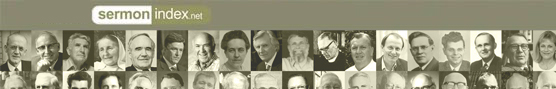Promoting Genuine Biblical Revival.
Sermon Podcast | Audio | VideoSermonIndex.net : Christian Books : PART FOURTH.--THE KINGDOM OF ISRAEL.

Hurlbuts Bible Lessons by Rev. Jesse Lyman Hurlbut

### PART FOURTH.--THE KINGDOM OF ISRAEL.

Lesson XXXII. The Two Kingdoms.

(Tell Stories 1 and 2 of Part Fourth.)

=1.= What took place after King S[)o]l´o-mon died? =The kingdom was divided.=

=2.= What was the larger part called? =The kingdom of [)I][s+]´ra-el.=

=3.= How many of the tribes were in the kingdom of [)I][s+]´ra-el? =Ten of the twelve tribes.=

=4.= Who was the first king of the Ten Tribes of [)I][s+]´ra-el? =J[)e]r-o-b[=o]´am.=

=5.= What was the smaller part called? =The kingdom of J[=u]´dah.=

=6.= What tribes were in the kingdom of J[=u]´dah? =J[=u]´dah and B[)e]n´ja-m[)i]n.=

=7.= Who was the first king of J[=u]´dah? =R[=e]-ho-b[=o]´am the son of S[)o]l´o-mon.=

=8.= What wicked thing did J[)e]r-o-b[=o]´am the king of [)I][s+]´ra-el do? =He led his people away from God.=

=9.= What did J[)e]r-o-b[=o]´am lead his people to do? =To pray to idols.=

=10.= What is J[)e]r-o-b[=o]´am called in the Bible? =|J[)e]r-o-b[=o]´am who made [)I][s+]´ra-el to sin.|=

=11.= What did the prophet of God tell J[)e]r-o-b[=o]´am? =That the kingdom should be taken from his family.=

Lesson XXXIII. Elijah.

(Read =11.= Where did he call down fire from heaven upon an altar? =On Mount Cär´mel.=

=12.= Where did God talk with [+E]-l[=i]´jah? =At Mount H[=o]´reb.=

=13.= How was [+E]-l[=i]´jah taken to heaven? =In a chariot of fire.=

=14.= Who was the prophet afte

<<  Contents  >>